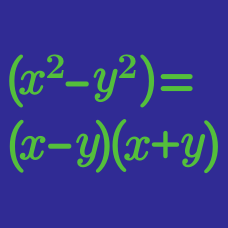Algebra

# Factoring Polynomials of Higher Degree

The polynomial $x^4-7x^3-76x^2-7x+1$ is factorized as $(x^2+ax+b)(x^2+cx+d),$ where $a$, $b$, $c$ and $d$ are real numbers and $a>0$. What is the value of $ad-bc$?

The polynomial $x^5-5x^4-31x^3-31x^2-5x+1$ is factorized as $(x+k)(x^2+ax+b)(x^2+cx+d),$ where $a$, $b$, $c$, $d$ and $k$ are constants and $a>0$. What is the value of $(a+k)d-bc$?

Suppose $f(x,y)$ and $g(x,y)$ are non-constant polynomials with integer coefficients in two variables, $x$ and $y$, such that $f(x,y)\cdot g(x,y)=x^3-3y^3+3x^2-xy^3$ Find $|f(3,3)|+|g(3,3)|.$

If $a$, $b$, $c$, $d$ and $e$ are non-negative integers such that

$x^6-289x^4-x^2+289=(x-a)(x+b)(x-c)(x+d)(x^2+ex+1),$

what is the value of $a+b+c+d+e$?

If $a$ and $b$ are positive numbers satisfying \begin{aligned} & x^4-5x^3+7x^2-43x+40 \\ &= (x-a)(x-b)(x^2+x+c), \end{aligned} what is the value of $a+b+c$?

×